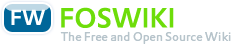# Course Theory of Imaging

Responsible: Prof. Dr. Stefan Altmeyer

## Course

### Meets requirements of following modules(MID)

• in active programs

### Course Organization

Version
created 2011-11-30
VID 1
valid from WS 2012/13
valid to
Course identifiers
Long name Theory of Imaging
CID F07_ABT
CEID (exam identifier)

Contact hours per week (SWS)
Lecture 2
Exercise (unsplit)
Exercise (split)
Lab 2
Project
Seminar
Tutorial(voluntary)
Total contact hours
Lecture 30
Exercise (unsplit)
Exercise (split)
Lab 30
Project
Seminar
Tutorial (voluntary)
Max. capacity
Exercise (unsplit)
Exercise (split)
Lab 15
Project
Seminar

Total effort (hours): 150

• Deutsch

• Bachelor

#### Prerequisites

• series expansion
• differential calculus
• integral calculus
• Fourier transfom
• geometrical optics
• wave optics

• Pedrotti, Pedrotti, Bausch, Schmidt: Optik für Ingenieure. Grundlagen (Springer)
• Hecht: Optik (Oldenbourg)
• Perez: Optik (Spektrum Akademischer Verlag)
• Goodman: Introduction to Fourier Optics (Roberts and Co. Publishers)
• Kurz, Lauterborn: Coherent Optics (Springer)

#### Instructors

• Prof. Dr. Stefan Altmeyer

• none

#### Transcipt Entry

Theory of Imaging

### Assessment

Type
oE normal case (except on large numbers on assessments: wE)

Total effort [hours]
oE 15

Frequency: 2-3/year

## Course components

### Lecture

#### Objectives

##### Lerninhalte (Kenntnisse)
• mathematics
• 2D Fourier transform
• linearity theorem
• similarity theorem
• shift theorem
• convolution theorem
• autocorrelation theorem
• Fourier transforms of special functions
• Hilbert space
• sckalar product
• norm
• basis vector representations
• completeness
• delta functionals
• definition in higher dimesnions, shifted
• sifting properties
• mathematically equivalent representations
• coherence
• representation as correlation function
• temporal coherence and Wiener-Chintschin theorem
• spatial coherence and Van-Cittert-Zernike theorem
• Theory of 2 dimensional linear systemsapplied to optical systems
• point spread function in amplitude and intensity
• optical transfer function in amplitude and intensity
• modulation transfer function
• phase transfer function
• relation to point spread function
• relation to pupil function
• relation to wavefront aberration function
• mathematical relation of coherent and incoherent optical transfer function
• coherent and incoherent frequency cutoff
• aberrations
• Seidel aberration
• phase representation in the exit pupil plane
• reason for the different aberrations
• strategies for aberration minimaziation
• Zernike polynomials
• measuring phases
• Shack-Hartmann sensor
• Shearing plate

##### Acquired Skills
• assured calculation of Fourier transforms with use of the Foutrier theorems
• analysis of optical systems
• identification of coherent and incoherent optical systens
• assured application of coherent and incoherent linear system theory
• identification and naming of aberrations
• design of optical setups for the measurement of phase and aberrations

• none

### Lab

#### Objectives

##### Acquired Skills
• using commerical software packages
• exploitation of aquired data
• graphical preparation of data

##### Operational Competences
• self-reliant planning and realization of optical setups
• measurement of point spread functions and optical transfer functions
• calculation of the point spread function from a given transfer function
• calculation of the transfer function from a given point spread function
• realization of a light source with adjustable degree of coherence
• measurement and interpretation of the transfer function of an objective in depedence on varying coherence
• measurement and interpretation of the modulation transfer funtion of an objective in dependence on a varying aperture stop
• writing of a scientific report
• precise description of the task
• representation of the approach
• description of the setup
• explanation of data expoitation
• presentation if the result and critical discussion

Type
fIN interview on specific topics
fTP supervised team project, groups as small as possible
fLP written presentation of outcomesDeutsch English